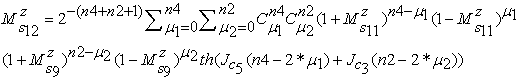﻿ Comparative Investigation and Generalized of the Core/Shell Effects on the Magnetics Properties in the Ferromagnetic Cubic Nanoparticles by the Transverse Ising Model

### Comparative Investigation and Generalized of the Core/Shell Effects on the Magnetics Properties in t...

Alioune Aidara Diouf, Bassirou Lo, Alhadj Hisseine Issaka Ali, Aboubaker Chedikh Beye

American Journal of Nanomaterials

## Comparative Investigation and Generalized of the Core/Shell Effects on the Magnetics Properties in the Ferromagnetic Cubic Nanoparticles by the Transverse Ising Model

Alioune Aidara Diouf1,, Bassirou Lo1, Alhadj Hisseine Issaka Ali1, Aboubaker Chedikh Beye1

1Groupe Physique des Solides et Sciences des Matériaux, Faculté des Sciences et Techniques, Université Cheikh Anta Diop de Dakar (UCAD), B.P. 25114, Dakar-Fann Dakar, Sénégal

### Abstract

The effective field theory within a probability distribution technique that accounts for the self-spin correlation functions is used to study the magnetic properties of the ferromagnetic system described by the spin S= 1/2 Ising model. The thermal behaviors of magnetization and longitudinal hysteresis are examined in details to study the effects of shell on the magnetics properties in the nanoparticles.

• Alioune Aidara Diouf, Bassirou Lo, Alhadj Hisseine Issaka Ali, Aboubaker Chedikh Beye. Comparative Investigation and Generalized of the Core/Shell Effects on the Magnetics Properties in the Ferromagnetic Cubic Nanoparticles by the Transverse Ising Model. American Journal of Nanomaterials. Vol. 4, No. 1, 2016, pp 1-7. http://pubs.sciepub.com/ajn/4/1/1
• Diouf, Alioune Aidara, et al. "Comparative Investigation and Generalized of the Core/Shell Effects on the Magnetics Properties in the Ferromagnetic Cubic Nanoparticles by the Transverse Ising Model." American Journal of Nanomaterials 4.1 (2016): 1-7.
• Diouf, A. A. , Lo, B. , Ali, A. H. I. , & Beye, A. C. (2016). Comparative Investigation and Generalized of the Core/Shell Effects on the Magnetics Properties in the Ferromagnetic Cubic Nanoparticles by the Transverse Ising Model. American Journal of Nanomaterials, 4(1), 1-7.
• Diouf, Alioune Aidara, Bassirou Lo, Alhadj Hisseine Issaka Ali, and Aboubaker Chedikh Beye. "Comparative Investigation and Generalized of the Core/Shell Effects on the Magnetics Properties in the Ferromagnetic Cubic Nanoparticles by the Transverse Ising Model." American Journal of Nanomaterials 4, no. 1 (2016): 1-7.

 Import into BibTeX Import into EndNote Import into RefMan Import into RefWorks

12
Prev Next

### 1. Introduction

The Surface of matters owns usually a chemical structure and physical properties different ones from matters consider in large number. The understanding of phenomenon linked to the surface of solids present a particular interest because of its position with the outside environment, but also because the states of surface can influence in the global properties of solids. In certain case, it's more the properties of shells than the ones of solids that we search to explore. We must to note the emergences of the researches in the nanomaterials give to the effects of shell on the magnetics properties, particularly exacerbating in these systems, a particular importance. The magnetic behavior of the particles of surface differs from that corresponding to the core because of the lower coordination number and the existence of broken exchange bonds. When we reduce the size of magnetic particles to the nanoscale, surface and finite-size effects play a dominant role on the magnetic properties. The increasing of the magnetics properties are often attributed to the frustration associated to the broken bonds and disorder present at the particle surface. Among the magnetic properties, the depending of the exchange interaction between spins in the particles and the geometry of their underlying lattice, surface spins may suffer random canting and these anomalous magnetic properties have been attributed to the existence of dipolar interactions among the particles. There are experimental evidences that surface effects and finite size are crucial in order to understand this phenomenology . Oscar and al. used maghemite (γ-Fe₂O₃) to study the effects of surface on the magnetic properties and use as magnetic properties the anisotropy  with the Monte Carlo simulation. In this study, we used another magnetic property, the exchange interaction; applied to the effective field theory within a probability distribution technique that accounts for the self-spin correlation functions to study the effects of the surface on the magnetic properties. To our knowledge, authors work on the antiferromagnetic nanocube with the separation of the core/shell spins, our works generalize the effects of surface on the cubic nanoparticles ferromagnetic. Our objective in this article is to study the effects of shell on the magnetics properties of a cubic nanoparticle considered as ferromagnetic with spins S=(1/2) within the framework of the effective field theory with probability distribution technique and generalized the results. This technique give acceptable results and the effects of the external longitudinal magnetic field on the magnetizations and hysteresis loops of the cubic nanoparticle lattice are discussed in detail in the case of the effects of core/shell.

### 2. Model and Formalism

We consider a system made up five layers with 125 spins; each layer has twenty-fives spins linked between them. The spins are regularly distributed on the surface of the layers and form a cubic Nano-system. The aim of this part is to model and define the effects of surface. The studies of the magnetics properties start by the count of the number of spin, which we will allow to establish the number of nearest neighbor of each spin to find the order parameter and deduct the magnetization and hysteresis. So, the counting of the number of spin shows 12 types, 6 types for the shell and 6 types for the core. The obtaining of the spins of shell and core, give us the opportunity to specify for each spin, the type and the number of spin used to establish the interaction between the nearest-neighbors. The Transverse Ising Model used is defined by:(1)

Or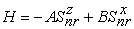Where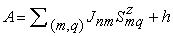and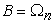. Withthe exchange interaction,andthe components following (Oz) and (Ox) of the spin S=1/2, (n,m) the index of the plans, (r,q) the index of the different sites of the plans (n,m) andthe transversal field.

From statistics of spin systems, for the operatorsat site n, one has:(2)

Where the angular bracket <...> denotes a canonical thermal average, β=1/kB.T, T is the temperature and α is the number of order parameter. If the exchange interactions are restricted to nearest-neighbors interactions only, the evaluation of the inner traces over selected spins in the last equations yields is(3)

Whereif r=z : Longitudinal andif r=x : Transversal

The above thermal averages are valid for a fixed spacial configuration. The step is to carry out the configurational averaging to be noted <...>r, the quantities of interest are the magnetizationdefined by: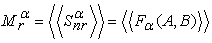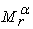is function depending oflike :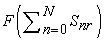. We introduce the Dirac delta function: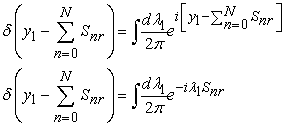(4)

We have: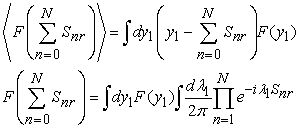(5)

In the approximation of Zernike decoupling of the multiple correlation, we have: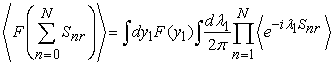(6)

To perform the configurational averaging on the right-hand side of the last equation, we use the probability distribution method based on the use of generalized Van der Wander identities. If this is done we have:(7)

Where the distribution functionsare given by: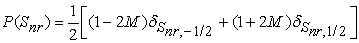(8)

Taking into account relations above, We get the following relation for the ordering parameters:(9)

In this equation, N represent the coordination number and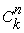is the binomial coefficients,. We have thus arrived at 12 self-consistent equations for the ordering parameters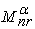with our following system used in this paper, that can be solved directly by numerical iteration without any further algebraic manipulations. This advantage of introducing the probability distribution technique. Then the average total magnetization is given by:(10)

With S: Shell and C: core, the details of calculation of the magnetization are given in the appendix.

The longitudinal susceptibility for the system can be determined from the following relation:A ferromagnetic cubic Nano-system (Figure 1) is used to study the effects of shell on the magnetic properties, taking into account relations above, With JS which represent the exchange interaction of shell, JC-S the exchange interaction of core-shell, JC the exchange interaction of core-core and C1,C2,C3 the core atoms for the plan 1, 3 (Figure 2) and C4,C5,C6 the core atoms for the plan 2 (Figure 2). We get the relations for the ordering parameters detailed in the appendix. We said in the beginning of this paper, the aim is to study the effects of shell on the magnetics properties. Therefore, we introduce three parameters in the equations of magnetization, which will be using to describe the effects of shell and core to compare the results. The parameters are called reduce exchange interaction, definite by:(11)

With rs : the reduce exchange interaction of shell, rcs : The reduce exchange interaction of core-shell, rc: The reduce exchange interaction of core-core, Js : The exchange interaction of shell, Jcs : The exchange interaction of core-shell and Jc : The exchange interaction of core-core.Download asVeiw figureFigures index
Figure 1. Ferromagnetic Cubic nanosystem, the black circles represent the atoms of the surface shellDownload asVeiw figureFigures index
Figure 2. Plan1, 2 and 3, the gray circles represent the magnetic atoms constituting the core and the black circles represent the atoms of the surface shell

### 3. Results and Discussions

The variation of the reduce exchange interaction show the particularity of the effects of shell on the magnetics properties as we saw on the curves of magnetization and hysteresis loops. The curves of magnetization obtained with our system shell-core, give approximates critical temperatures because of their types of spin and the coordination number, this phenomenon was noted on shell-core maghemite nanoparticles γ-F₂O₃ . For values of kB.T/Jc = 0 (M= 0.5 for rs = 0.5, 1.0 and 2.0), the maximum of magnetization observed on the Figure 3 is characteristic of the ferromagnetic cubic nanoparticles because of the orientation of the spins in the same direction linked by a high exchange interaction, one among the magnetic properties responsible of the magnetization, when the spins aren't submitted to an exterior excitation. From kB.T/Jc =0.5 (case where rs=1.0), the magnetization which was stable before, start to decrease and converge to a new value of the temperature which define the critical temperature of the system. The temperature kB.T/Jc =0.5 being used as starting point of the falling of the magnetization toward the critical temperature (TC ), coincides with the blocking temperature (TB) of the system which defined the beginning of the super-paramagnetism between TB and TC observed in the ferromagnetic nanoparticles. And the same thing is observed for the other curves of magnetization on the Figure 3 when (rs), the reduce exchange interaction, increase from 0.5 to 2.0. Moreover, we observe the same situation on the Figure 4 which show us the magnetizations with variation of the reduce exchange interaction core-shell (rcs), where for values of kB.T/Jc = 0 we have a maximum magnetization with M= 0.5, beginning point of all the curves of magnetization, even with a variation of rcs. We observe in the case where rcs=0.5, we have a correct curve of magnetization characteristic of the ferromagnetic nanoparticles with a critical temperature kB.T/Jc = 1.27 and from rcs=1.0 to 2.0, we observe an approximate critical temperature as in the case of the Figure 3.Download asVeiw figureFigures index
Figure 3. Curve of magnetization with the changing of the reduce exchange interaction of Shell (rs)Download asVeiw figureFigures index
Figure 4. Curve of magnetization with the changing of the reduce exchange interaction of Core-Shell (rcs)

The Figure 5 and Figure 6 give information about the hysteresis loops we observe that in the Figure 5 more the exchange interaction of shell (rs) increase more the shape of the hysteresis loops change and the parameters are important. With the changing of the reduce exchange interaction of shell (rs) from 0.5 to 2.0, kB.T/Jc = 1.0 and ω=1.0 the hysteresis loops are symmetric with respect to the zero-magnetic field axis. We notice that, when we increase the surface exchange coupling from 0,5 to 2.0, the shape of the central loop changes from the round to square. The hysteresis loops observed in the Figure 6, with the changing of the reduce exchange interaction of core/shell (rcs) from 0.5 to 2.0, kB.T/Jc = 1.0 and ω=1.0 show a symmetric shape with the magnetization curves unless the fig. 5 even if we observe the respect of the zero-magnetic field axis in the fig. 6 we have a superposition of the various hysteresis loops from rcs=0.5 to 2.0. The last observation, show us the influence of the external excitation on the hysteresis loops of shell and of core as we saw on the Figure 5 and Figure 6. That's way the most important of the magnetizations are done on the shell as we see on the Figure 3 and Figure 5 and the other part obtained with the nanoparticle of core are obtained with the reduce exchange interaction core/shell.Download asVeiw figureFigures index
Figure 5. Hysteresis loop with the changing of the reduce exchange interaction of Shell (rs)Download asVeiw figureFigures index
Figure 6. Hysteresis loop with the changing of the reduce exchange interaction of Core-Shell (rcs)

### 4. Conclusion

In this work, we studied the effects of shell on the magnetics properties by using Transverse Ising Model. And we observe, the influences of the shell parameters are more important than the influences of the core-shell parameters. We deduced, the important part of the magnetization is done on the surface, so the curves of magnetization and the hysteresis loops show us, the different between the effects of shell and of core. The approximate critical temperature observed in the curves of magnetization of the core/shell system was noted by the authors on the maghemite nanoparticles γ-Fe₂O₃ , and these paper demonstrate and generalized the results on the effects of shell on the ferromagnetic cubic nanoparticles with the none separation of the parameters of core and shell as we saw with certain authors . In the next article, with the same concepts, one will show the applications of the electric and magnetic field on the ZnO nanoparticles of type wurtzite to study the polarization and the magnetic properties as the curves of susceptibility.

### Acknowledgment

Pr. Beye gratefully acknowledges for your hospitality in your Nanoscience Laboratory at the University Cheikh Anta Diop of Dakar where i performed these results and where i continue my researches for my PhD.

### References

  S. Bouhou, I. Essaoudi, A. Ainane, M. Saber, F. Dujardin, J.J. de Miguel, Journal of magnetism and Magnetic Materials 324, 2434(2012).In article View Article  S. Bouhou, I. Essaoudi, A. Ainane, M. Saber, R.Ahuja, F. Dujardin, J. Supercond Nov Magn. 26, 201(2013).In article View Article  Oscar Iglesias, Amilcar Labarta, Journal of Magnetism and Magnetic Materials 738,290 (2005).In article  Oscar Iglesias, Amilcar Labarta, Physica B 343, 286 (2004).In article View Article  M. El Hamri, S. Bouhou, I.Essaoudi, A. Ainane, R. Ahuja, Investigation of the surface shell effects on the magnetic properties of a transverse antiferro-magnetic Ising model, Superlattices and Microstructures S0749-6036(15)00006-3 (2015).In article

### Appendix

The magnetizations for each spin (S: Shell and C: Core):

A)- S1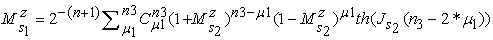B)- S2C)- S3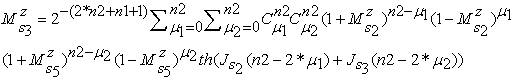D)- S4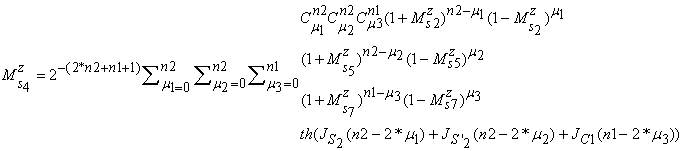E)- S5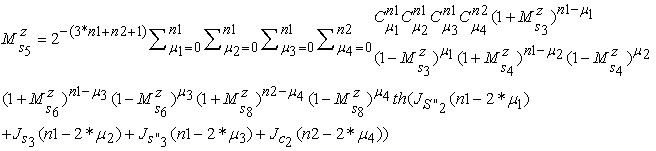F)- S6G)- C1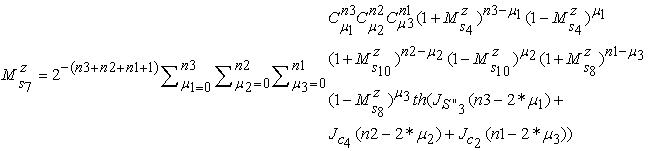H)- C2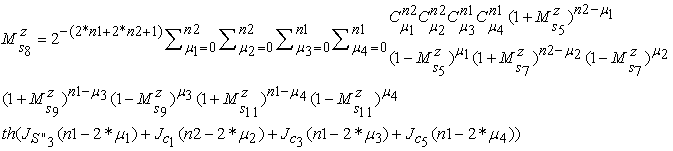I)- C3J)- C4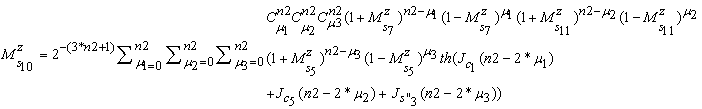K)- C5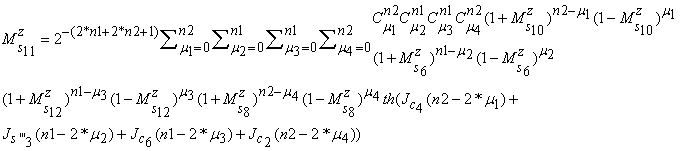L)- C6